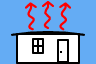http://people.csail.mit.edu/jaffer/SimRoof/Convection

# Convection From a Rectangular Plate

## Abstract

New research into convection yields practical formulas sufficient to calculate roof convection from roof parameters and meteorological data.

Through the use of effective-characteristic-length, the model is extended to natural convection for all orientations of a plate, not just those having a horizontal edge, off-axis forced (but in-plane) flows, and forced convection averaged over all in-plane directions of flow.

A related model for convection from symmetrical peaked roofs is also developed.

## Introduction

### Motivation

The most important thermal processes for a building's roof are absorption of solar radiation (insolation), convection, and thermal radiation.

Currently, established theory is not sufficient to compute convection from roof parameters and meteorological data. This work rectifies that situation.

### Convective Heat Transfer

Says Wikipedia:

Convective heat transfer, often referred to simply as convection, is the transfer of heat from one place to another by the movement of fluids.

To model the thermal dynamics of roofs we are interested in the convective flow of heat to or from the top surface of the roof. For a flat plate at a uniform temperature T, the rate of heat flow (in Watts) due to convection from one side of the plate is:

 h ⋅ A ⋅ ΔT h W/(K⋅m2) convective surface conductance A m2 area of one side of plate ΔT K T − Tenv T K plate temperature Tenv K fluid temperature

The complication is that the value of h depends on temperatures, fluid-velocity, and the area, shape, orientation, and roughness of the plate surface. A value of h for a 1 m by 1 m plate will usually be larger (and never smaller) than h for a 2 m by 2 m plate under otherwise identical conditions. The larger plate will transfer more heat because it has four times the area of the smaller plate, but not more than 4 times the heat.

### Types of Convection

Convection is natural (free), forced, or mixed. In natural convection, fluid motion is driven by the difference in temperature between the plate and the fluid. In forced convection, fluid motion is driven by an external force such as wind. Mixed convection is a mixture of natural and forced convection occurring at low fluid speeds.

(Natural) upward convection is produced by an upward-facing plate which is warmer than the fluid, or a downward-facing plate which is colder than the fluid. (Natural) downward convection is produced by an upward-facing plate which is cooler than the fluid, or a downward-facing plate which is warmer than the fluid.

A correlation (approximate equation relating dimensionless quantities) can model an isothermal surface or a constant heat flux through the surface. The correlations for these two regimes usually differ only in coefficients and additive constants. This article addresses convection from isothermal plates.

A forced convection formula can be for local heat flow or for heat flow averaged across the surface. Section "Laminar-Turbulent Progression" gives the derivation for average heat flow from the local heat flow which appears in several texts. While it produces the customary averaged formulas for purely laminar or purely turbulent flow, the formula it produces for transitional flows requires a Reynolds-number threshold from measurement of the specific configuration under investigation, which limits its predictive ability.

Convection formulas can be derived from theory, numerical simulation, or experiment (empirical). The accepted formulas for natural convection are empirical.

At the plate surface, the fluid velocity is near zero. At some distance from the plate surface the fluid velocity approaches the bulk fluid velocity. In between is the boundary layer. Flow in the boundary layer is laminar or turbulent. The h values for turbulent regions of the plate (boundary layer) are larger than h values for laminar regions. Natural upward convection (from a horizontal plate) transitions from laminar to turbulent at Rayleigh numbers (Ra) near 107 (Clear et al), placing it between the ranges for formulas T9.7 and T9.8 (which intersect at 4.7×106). Convection from a vertical plate has a transition around Gr=Ra/Pr=109.

Natural convection is strongly affected by the inclination of a plate. The natural convective surface conductivity from a vertical plate is between the conductivities of upward facing and downward facing level plates of the same size. Upward convection has the largest heat flow. Forced convection is insensitive to inclination.

Forced convection is greater for rough surfaces than for smooth ones. Natural convection is insensitive to roughness whose mean height is much less than the dimensions of the plate.

## Parameters

### Physical Parameters

symbol units description TF K Fluid (Air) Temperature TS K Plate Surface Temperature T K Mean Temperature (TF+TS)/2 P Pa Fluid (Atmospheric) Pressure V m/s Fluid Velocity Rair J/(kg⋅K) Gas Constant for Dry Air = 287.058 J/(kg⋅K) Φ Pa/Pa Relative Humidity

From these parameters are derived density ρ, thermal conductivity k, specific heat cp, and viscosity μ. For dry air, Kadoya, Matsunaga, and Nagashima seems the authoritative source for viscosity and thermal conductivity.

symbol forced natural formula units description cp ✓ ✓ [see text] J/(kg⋅K) specific heat at constant pressure ρ ✓ ✓ P/(Rair ⋅ T) kg/m3 density k ✓ ✓ [see text] W/(m⋅K) thermal conductivity μ ✓ ✓ [see text] Pa⋅s dynamic viscosity ν ✓ ✓ μ/ρ m2/s kinematic viscosity α ✓ k/(ρ⋅cp) m2/s thermal diffusivity β ✓ 1/T K−1 coefficient of thermal expansion

The moist air values of these properties are computed by combining the values for dry air and water vapor in proportion to their presence in the moist air mixture, in some cases with correction factors. What is true of all the mixture formulas is that at 0% relative humidity the mixture values are identical with the dry air values and at 100% relative humidity at 100°C the mixture values are identical with the steam (water vapor) values.

These mixture formulas come from Tsilingiris and Morvay and Gvozdenac (not ASHRAE). Both sources contain errors; the obvious errors don't occur in the corresponding quantities. Wexler seems the authoritative source for water-vapor (partial) pressure versus temperature.

### Thermodynamic and Transport Properties of AirAt 100% relative humidity, the pressure and mass fraction of vapor increase with temperature, but remain less than 10% at 45°C. So humidity will not be a major influence on air's properties at outdoor temperatures.

Model moist air as a mixture of ideal gases. The relative-humidity, Φ, is the ratio of the partial-pressure of water vapor to Psat. Psat is the partial pressure of saturated water vapor at temperature TF (Kelvins):

 Psat = 610.78 ⋅ 107.5 (TF−273.15)/(TF−35.85)

Over the temperature range of interest, this formula for Psat is well within 1% of the values returned by formula 16b in Wexler:

 Psat = exp( −0.63536311×104/TF +0.3404926034×102 −0.19509874×10−1⋅TF +0.12811805×10−4⋅TF2 )

Density ρ, thermal conductivity k, specific heat cp, and viscosity μ are computed at temperature T, the average of TF and TS. But Psat must be evaluated at the bulk fluid temperature TF because the amount of water-vapor in the fluid doesn't change when heated to intermediate temperature T. If TS is colder than TF, then condensation may occur.

Simulation of roofs can side-step the issue by constraining the roof temperature to not drop below the ambient dew-point temperature. Because of water's high latent heat, this treatment should not result in large errors.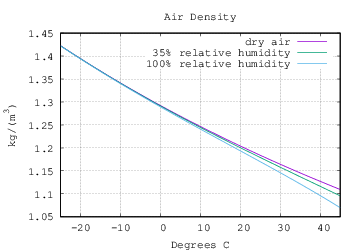For an ideal gas, density ρ is:

 ρ=P M/(R T) R=8.314 J/(kg⋅mol)

Model moist air as a mixture of dry air and water vapor:

 ρ = (P − Φ Psat) Ma + Φ Psat Mv R T R = 8.314 J/(kg⋅mol) Ma = 0.028964 kg/mol Mv = 0.018016 kg/molThe specific heat at constant pressure cp for dry air and water vapor each vary little over our temperature range. But the mixture at a given relative humidity is sensitive to temperature. cpa and cpv are the specific heat of air and water-vapor, respectively. These formulas from Tsilingiris take temperature t in degrees Celsius. xv(T) takes temperature in Kelvins.

 cpa(t) = 1034 −.2849⋅t +.7817×10−3⋅t2 −.4971×10−6⋅t3 +.1077×10−9⋅t4 cpv(t) = 1869−2.578×10−1⋅t +1.941×10−2⋅t2
 cp= cpa(t) (1−xv) Ma + cpv(t) xv Mv (1−xv) Ma + xv Mv xv(T) = Φ Psat(T)/P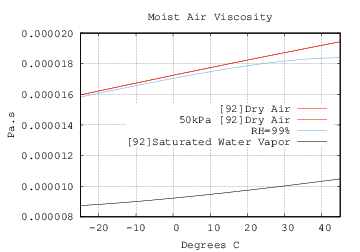Both  and  give formulas for the viscosity of dry air.  matches the data from  better; the values from  are about 1% lower; but result in (lower) Pr values closer to those from many sources. The formula from  is:

 μa = −9.8601×10−7 +9.080125×10−8⋅T −1.17635575×10−10⋅T2 +1.2349703×10−13⋅T3 −5.7971299×10−17⋅T4

The formula from  is:

 μa = −0.40401×10−6 +0.074582×10−6⋅T −5.7171×10−11⋅T2 +2.9928×10−14⋅T3 −6.2524×10−18⋅T4

A line for the viscosity at half air pressure (P=50 kPa) overlays Dry air; so air pressure variations don't significantly affect viscosity at roof conditions.

The viscosity of saturated water vapor is less studied. Tsilingiris gives a formula which returns a viscosity value for 100 C around half of that shown in his own graph of viscosity! Morvay and Gvozdenac give a formula for the viscosity of water vapor which matches Tsilingiris' graph well. With γ=647.27/T:

 μv = γ−1/2 018158.3 + 017762.4 γ + 010528.7 γ2 −003674.4 γ3

Over the temperature range of interest, μv is hardly different from:

 μv = 9.2173×10−6 Pa⋅s + (T − 273.15 K) ⋅ 25.713×10−9 Pa⋅s/K

Morvay and Gvozdenac introduce a parameter they call absolute humidity, the ratio of masses of water vapor and dry air:

 χ= Mv Psat Ma (P−Psat)

The dynamic viscosity of the moist air mixture is:

 μ = μa 1 + ΦAV ⋅ χ + μv 1 + ΦVA / χ

The terms ΦAV and ΦVA are complicated expressions having values ranging from 1.064 to 1.073 and .93 to .923 respectively over the roof range of interest (-25°C to 45°C).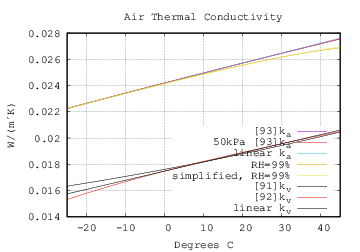Kadoya, Matsunaga, and Nagashima seems an authoritative source for the thermal-conductivity of dry air:

 ρr = P / (287.058 ⋅ 314.3 ⋅ T) Tr = T / 132.5 ka = 0.0259778 ⋅ ( 0.239503⋅Tr +0.00649768⋅Tr1/2 +1.0 −1.92615⋅Tr−1 +2.00383⋅Tr−2 −1.07553⋅Tr−3 +0.229414⋅Tr−4 +0.402287⋅ρr +0.356603⋅ρr2 −0.163159⋅ρr3 +0.138059⋅ρr4 −0.0201725⋅ρr5 )

A line for the k at half air pressure (P=50 kPa) overlays Dry air on the graph; so air pressure variations don't significantly affect k at roof conditions. Over the roof range of interest this is hardly different from:

 ka = 0.02241 W/(m⋅K) + (T−250 K) ⋅ 76.46×10−6 W/(m⋅K2)

Tsilingiris gives a formula for the thermal conductivity of water vapor:

 kv = 1.761758242×101 +5.558941059×102 T +1.663336663×104 T2

Morvay and Gvozdenac also give a formula for the thermal conductivity of water vapor:

 kv = 1.74822×10−2 +7.69127×10−5 t −3.23464×10−7 t2 +2.59524×10−9 t3 −3.17650×10−12 t4

They diverge mostly at the dry end of the curve, where kv has insignificant effect on the moist air thermal-conductivity (km). Over the roof range of interest the latter curve is hardly different from:

 kv = 0.0174822 W/(m⋅K) + (T−273.15 K) ⋅ 69.4587305×10−06 W/(m⋅K2)

Morvay and Gvozdenac's formula for moist air thermal conductivity is like the formula for viscosity, but with more complicated expressions for ΦAV and ΦVA. Tsilingiris's formula uses the same ΦAV and ΦAV as the viscosity formula:

 k = ka 1 + ΦAV ⋅ χ + kv 1 + ΦVA / χ

The simplified 99% RH curve, which is nearly identical to the 99% RH curve, uses the linear ka and kv models and substitutes 1.07 and 0.93 for ΦAV and ΦVA.For an ideal gas with pressure held constant, the volumetric thermal expansivity (i.e. relative change in volume due to temperature change) is the inverse of temperature. For natural convection from a horizontal plate it is:

β=1/T

For natural convection from a vertical plate (T9.2, Nu') it is:

β=1/TF

### Characteristic Length

For a flat rectangular plate, Lc is the length of the side parallel to the direction of flow.

For a flat plate, L* is the area (of one side) of the plate divided by its perimeter. Here are formulas for L* of four shapes. l is the length; w is the width; D is the diameter.

 rectangle square infinite strip circular disk L*(w, l) = w⋅l 2 (w+l) L*(w, w) = w 4 L*(w, ∞) = w 2 π D2 / 4 π D = D4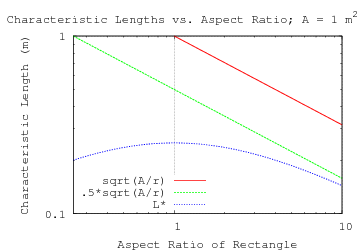It is interesting that a square and the maximal circle inscribed within it have the same L*.

The plot to the right shows how the length of a side (sqrt(A/r)) and L* (area/perimeter) vary with aspect-ratio for a rectangular plate with an area of 1 m2. As the aspect-ratio r grows, L* tends to sqrt(A/r)/2.

The hydraulic-diameter, used as the characteristic length DH in ducts, is related to L*, being 4 times the cross-section area divided by the cross-section perimeter.

### Dimensionless Quantities

Below are formulas for dimensionless quantities governing convection along with ranges for air under the conditions:

Dry-Bulb Temperature
between −25°C and +45°C
Temperature difference between the roof and dry-bulb
between −10 K and +50 K
Atmospheric pressure
between 88300 Pa and 103600 Pa
Relative humidity
between 0% and 100%
Wind Speed
between 1 m/s and 15 m/s
Plate size
between 1 m and 50 m length; between 1 m and 50 m width
Root-mean-squared height of roughness ε
between 0 mm and roof side / 300.
gravitational acceleration g
9.80665 m/s2

Ranges for vertical plates, where Lc is the height, are marked with an apostrophe ('). Ranges for horizontal upward-facing plates, where L* is the ratio of area to perimeter, are marked with an asterisk (*). Ranges for horizontal downward-facing plates, where LR is half the length of the shorter side, are marked with a subscript R. The Prandtl number is insensitive to L, depending only on fluid (air) properties. The Lc for Reynolds and Nusselt numbers in forced convection is the length of the plate in the direction of flow.

Both Gr and Ra have factors of |ΔT|; they will be zero when ΔT is zero. In order to get an idea of the dynamic range of Gr and Ra, the minimum ΔT used for range-of-interest is 1 K rather than 0 K. Re will be zero when the wind-speed is zero. In order to get an idea of the dynamic range of Re, the minimum V used for range-of-interest is 1 m/s rather than 0 m/s.

In convection calculations, Nu is computed from the other dimensionless quantities; then solved for h, the surface conductance (having units W/(m2⋅K)).

symbol formula expansion roof range of interest description Pr ν/α cp⋅μ/k 0.697≤Pr≤0.729 Prandtl number Gr β⋅|ΔT|⋅g⋅Lc3ν2 |ΔT|⋅g⋅Lc3⋅ρ2T⋅μ2 Grashof number for natural convection Ra Gr⋅Pr |ΔT|⋅g⋅Lc3⋅ρ2⋅cpT⋅μ⋅k 9.34×106≤Ra*≤1.37×1013 5.97×108≤Ra'≤8.74×1014 7.47×107≤RaR≤1.09×1014 Rayleigh number for natural convection Re V⋅Lc/ν V⋅Lc⋅ρ/μ 4.40×104≤Re≤7.15×107 Reynolds number for forced convection Nu RSTT8.9, T8.11 (forced rough)(forced smooth) 2.00×102≤Nur≤3.28×105 1.70×102≤Nus≤6.36×104 Nusselt number Nu*Nu'NuR (natural) 8.70≤Nu*≤3.45×103 1.05×102≤Nu'≤1.10×104 21.9≤NuR≤4.85×103 h Nu⋅k/Lc (forced rough)(forced smooth) 5.87≤hr≤4.78×102 2.90≤hs≤1.59×102 convective surface conductance W/(m2⋅K) Nu⋅k/L* Nu⋅k/L' Nu⋅k/LR (natural) 0.189≤h*≤7.62 2.46≤h'≤5.83 0.259≤hR≤4.98

It is worth noting that a larger h value doesn't necessarily correspond to a larger Nu value because Nu gets divided by different L values.

## Natural Convection

From Thermodynamic Basis for Natural Convection from an Isothermal Plate the formulas for upward facing, vertical, and downward facing plates are:

 Nu*(Ra*) = ( 0.671 + 0.370 Ra*1/6 )2 (Nu*) Nu'(Ra') = 0.682 ( 1 + 0.469 [Ra' / Ξ(Pr)]1/6 )2 (Nu') NuR(RaR) = 0.682 + 0.550 [RaR / Ξ(Pr)]1/5 (NuR) Ξ(Pr) = ‖ 1, 0.5 / Pr ‖1/√3 ‖ x, y ‖p = ( xp + yp )1/p

These formulas are combined to yield natural convection from a flat plate inclined at angle θ:

 h = k max( Nu'(Ra' |cos θ|) / L', Nu*(Ra* |sin θ|) / L* ), if ΔT sinθ < 0; h = k max( Nu'(Ra' |cos θ|) / L', NuR(RaR |sin θ|) / LR ), otherwise.

The upward characteristic-length L* is the area to perimeter ratio. The vertical L' is the length of the rectangle's vertical edge. The downward LR is half of the rectangle's shortest edge.

## Forced Convection

The common formulas for forced convection from a smooth flat plate are due to Blasius' 1908 mathematical analysis.

Recalling Dimensionless Quantities, we introduce local versions of Nu and Re:

symbol formula expansion description Nux hx⋅x/k local Nusselt number Nu h⋅Lc/k average Pr ν/α cp⋅μ/k fluid Prandtl number Rex V⋅x/ν V⋅x⋅ρ/μ local Reynolds number Re V⋅Lc/ν V⋅Lc⋅ρ/μ average

The table below excerpts those sections of Table 8 from chapter 4 of 2009 ASHRAE Fundamentals Handbook (SI) which deal with convection from flat plates. The plate orientation is not specified. L is the length of the plate [assumed in the direction of fluid flow]. No citations are given for these 5 formulas. Rec does not appear in the text.

 III. External Flows for Flat Plate: Characteristic length = L = length of plate. Re = VL/ν. All properties at arithmetic mean of surface and fluid temperatures. Laminar boundary layer: Re<5×105 Nu = 0.332 Re1/2 Pr1/3 Local value of h (T8.8) Nu = 0.664 Re1/2 Pr1/3 Average value of h (T8.9) Turbulent boundary layer: Re>5×105 Nu = 0.0296 Re4/5 Pr1/3 Local value of h (T8.10) Turbulent boundary layer beginning at leading edge: All Re Nu = 0.037 Re4/5 Pr1/3 Average value of h (T8.11) Laminar-turbulent boundary layer: Re>5×105 Nu = (0.037 Re4/5−871) Pr1/3 Average value Rec=5×105 (T8.12)

Chapter 4 of 2009 ASHRAE Fundamentals states:

For a flat plate with a smooth leading edge, the turbulent boundary layer starts at distance xc from the leading edge where the Reynolds number Re=V⋅xc/ν is in the range 300000 to 500000 (in some cases, higher). In a plate with a blunt front edge or other irregularities, it can start at much smaller Reynolds numbers.

At 15°C, the kinetic viscosity of air is ν=15.7 ×10−6 m2/s. At V=10 m/s and Re=4×105, xc=Re⋅ν/V=.63 m. At V=2.5 m/s xc=2.5 m. The higher wind speeds will induce a mixture of laminar and turbulent convection on smooth panels larger than 1 m.

### Laminar-Turbulent Progression

Local Re changes with distance (x) from the leading edge of the plate. Casting T8.8 and T8.10 in terms of h and x:

 hL = 0.332 k⋅(x⋅V/ν)1/2 Pr1/3x laminar x⋅V/ν<5×105 hT = 0.0296 k⋅(x⋅V/ν)4/5 Pr1/3x turbulent x⋅V/ν>5×105

The boundary position, xc, is the minimum of Lc and 5×105 ν/V.

 xc = min(Lc, 5×105 ν/V)

Integrate along the direction of flow from leading edge to Lc; then divide by Lc to obtain the average value of h for the plate.

 h = 1Lc ( xc∫ 0 hL(x) dx + Lc∫ xc hT(x) dx ) = k ⋅ Pr1/3Lc ( xc∫ 0 .332 (V/ν)1/2 x−1/2 dx + Lc∫ xc .0296 (V/ν)4/5 x−1/5 dx ) = k ⋅ Pr1/3Lc ( 0.664 (V/ν)1/2 ⋅ xc1/2 + 0.037 (V/ν)4/5 ⋅ (Lc4/5−xc4/5) ) (FH)

FH can be cast as a dimensionless formula by reversing the previous substitutions:

 Nu = 0.664 Rec1/2 Pr1/3 + 0.037 (Re4/5−Rec4/5) Pr1/3 (FC)

When Rec=5×105, T8.12 results. When the flow is entirely laminar, xc=Lc, and T8.9 results. If the impinging flow is turbulent, then the boundary layer is all turbulent, xc=0, and T8.11 results.

## Surface Roughness

Skin-Friction and Forced Convection from an Isothermal Rough Plate gives a formula for forced convection from isothermal plates having self-similar or periodic roughness in terms of root-mean-squared (RMS) height-of-roughness ε > 0:

 Nu = Re Pr1/3 6 ln2(L/ε) L ε ≫ 1 (RT)

Dimensional analysis indicates that for periodic isotropic roughness with period LS ≪ L, the least upper bounds for laminar and smooth-turbulent flow along the plate will be, respectively:

 ReL = 0.6642 L LS 2 ε2 ReS = 0.0365 L LS4 24 ε5

When LS/ε < 388, the flow should transition directly from laminar to rough-turbulence at ReL. When LS/ε > 388, the flow should transition from laminar to smooth-turbulence at critical Reynolds number ReL, and to rough-turbulence at ReS.

Not having a definitive laminar-turbulent threshold for the smooth plate substantially reduces predictive value. Every plate is a rough surface at some scale. If the profile roughness is weakly isotropic and has an effective roughness repeat length LS ≪ L, then min(ReS,ReL) can take the place of Rec. L/LS=15 and L/ε=5800 results in a critical ReL=5×105.

Recasting ReL and ReS as functions of Re adapts formula (FC) to all three flow regimes:

 ReL = min(Re, 0.6642 L LS 2 ε2 ) ReS = min(Re, 0.0365 L LS4 24 ε5 )

When LS/ε < 388:

 Nu Pr1/3 = 0.664 ReL1/2 + Re−ReL 6 ln2(L/ε)

When LS/ε > 388:

 Nu Pr1/3 = 0.664 ReL1/2 + 0.037 (ReS4/5−ReL4/5) + Re−ReS 6 ln2(L/ε) (FCC)

## Off-Axis Convection

Over flat plates, forced fluid flow is assumed to have straight, parallel streamlines. Thus convex plates with non-uniform characteristic-length can be analyzed by summing the results of the plate partitioned into strips which are parallel to the flow.

### Forced Off-Axis Convection

Generalizing the analysis at the end of Laminar Convection finds that the ratio between hd (diagonal) and hp (axis-aligned) is 2(1+E)/2/(1+E) where E is the exponent of Re. For forced turbulent convection (E=4/5), sensitivity to rotation of the square is less than 4%.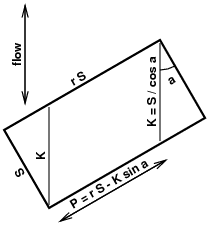Most roofs aren't square. Consider a S by rS rectangular plate with flow in the plane of the plate at angle φ from a length S side. For 0≤φ<π/2 and r≥tan φ, the strips which run between opposite rS sides have length K=S/cos φ. The base of the parallelogram is P=rSK⋅sin φ. The width of the parallelogram perpendicular to the flow is P⋅cos φ.

To find the average surface conductance for the whole plate, sum the product of the area of each strip and its average h (using its length as L), then divide by the total area rS2:

 ( h(K) K P cos φ + 2 K cos φ sin φ∫0 h( w cos φ sin φ ) w cos φ sin φ dw ) / (r S2)
 = .037 k Pr1/3 (V/ν)E ( KE P cos φ + 2(cos φ sin φ)E K cos φ sin φ∫0 wE dw ) / (r S2)
 = .037 k Pr1/3 (V/ν)E ( KE P cos φ + 2 K1+E1+E cos φ sin φ ) / (r S2)
 = .037 k Pr1/3 (V/ν)E (S/cos φ)E S/cos φ ( 1 + 1−E1+E ⋅ tan φr )
 = .037 k Pr1/3 (V/ν)4/5 (S/cos φ)−1/5 ( 1 + tan φ9 r )When 0<r≤tan φ (and 0≤φ<π/2), the strips which run between opposite S sides have length K=rS/sin φ. The base of the parallelogram is P=SK⋅cos φ. The width of the parallelogram perpendicular to the flow is P⋅sin φ. The average surface conductance for the whole plate is:

 ( h(K) K P sin φ + 2 K cos φ sin φ∫0 h( w cos φ sin φ ) w cos φ sin φ dw ) / (r S2)
 = .037 k Pr1/3 (V/ν)E ( KE P sin φ + 2(cos φ sin φ)E K cos φ sin φ∫0 wE dw ) / (r S2)
 = .037 k Pr1/3 (V/ν)E ( KE P sin φ + 2 K1+E1+E cos φ sin φ ) / (r S2)
 = .037 k Pr1/3 (V/ν)E (r S/sin φ)E r S/sin φ ( 1 + 1−E1+E ⋅ rtan φ )
 = .037 k Pr1/3 (V/ν)4/5 (r S/sin φ)−1/5 ( 1 + r9 tan φ )

For every rectangle with r>1, there is an identical (but rotated 90°) rectangle with S'=rS and r'=1/r. Taking the geometric mean of the side lengths r1/2S as the characteristic length L allows these expressions to be written as the standard forced convection surface conductances (derived from T8.11 and T8.9) times rotation factors Ftur(rφ) and Flam(rφ):

 h = .037 k Pr1/3 (V/ν)4/5 L1/5 Ftur(r, φ) HTRR
 Ftur(r, φ) = |cos φ|1/5 r1/10 ( 1 + |tan φ|9 r ) r>|tan φ| FTRR ( |sin φ|1/5 / r1/10 ) ( 1 + r9 |tan φ| ) 0
 h = .664 k Pr1/3 (V/ν)1/2 L1/2 Flam(r, φ) HLRR
 Flam(r, φ) = |cos φ|1/2 r1/4 ( 1 + |tan φ|3 r ) r>|tan φ| FLRR ( |sin φ|1/2 / r1/4 ) ( 1 + r3 |tan φ| ) 0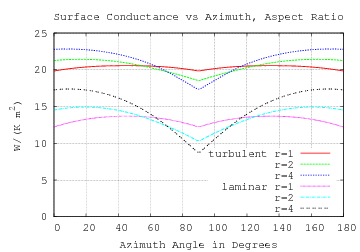To the right are shown the surface conductances for smooth (equal area) flat plates .35 m by .35 m, .5 m by .25 m, and .7 m by .175 m versus azimuth angle of the forced air flow (3.4 m/s at 21°C). At an azimuth of 0° the flow is parallel to a shortest side.

Both turbulent and laminar conductances are shown. The dips in the turbulent h will probably not be as sharp as shown due to the directional fluctuations in a turbulent flow.

The corresponding formulas are T8.11 and T8.9 times Ftur(rφ) and Flam(rφ) (with L = r1/2S):

 Nu = .037 Pr1/3 Re4/5 Ftur(r, φ) CTRR Nu = .664 Pr1/3 Re1/2 Flam(r, φ) CLRR

This is a mathematical derivation; I know of no in-plane off-axis forced-convection experiments.

That Ftur and Flam are independent of Re and L is a useful result. Ftur and Flam will be used to find h averaged over all azimuth angles in the next section.

The shape factor for the rough-surface formula RS is more difficult to compute because it doesn't have a simple power dependency on L.

For r≥|tan φ|:

 K = S / |cos φ| P = r S−K |sin φ| = S (r − |tan φ|)

The average surface conductance for the whole plate is:

 hav = ( h(K) K P |cos φ| + 2 K |cos φ sin φ|∫0 h( w |cos φ sin φ| ) w |cos φ sin φ| dw ) / (r S2)
 = h(K) (1 − |tan φ| / r) + 2r S2 S |sin φ|∫0 h( w |cos φ sin φ| ) w |cos φ sin φ| dw

When 0<r≤|tan φ|:

 K = r S / |sin φ| P = S−K |cos φ| = S (1 − r / |tan φ|)

The average surface conductance for the whole plate is:

 hav = ( h(K) K P |sin φ| + 2 K |cos φ sin φ|∫0 h( w |cos φ sin φ| ) w |cos φ sin φ| dw ) / (r S2)
 = h(K) (1 − r / |tan φ|) + 2r S2 r S |cos φ|∫0 h( w |cos φ sin φ| ) w |cos φ sin φ| dwThe solid lines in the graph to the right show the rotation-factors for 1 m2 rectangular plates with ε=10−4 m and aspect-ratios of 1:1 (black), 2:1 (blue), and 4:1 (red).

### Effective Length

Clear et al give characteristic-length values of L=28.3 m and L*=10.2 m. The roof they measured was neither round nor rectangular, yet they find that meteorological wind direction doesn't correlate with turbulent convection from that flat horizontal surface:

None of the data showed any correlation with wind direction, so this was not included in any of the fits.

This could either be because surface condutivities of different wind directions on their roof are close in value; or because wind direction is so variable within their 15-minute sampling intervals that the variations mostly average out. The only information Clear et al give about the roof dimensions is area (2940 m2), perimeter (287 m), and mean distance of the perimeter from the measurement point (28.3 m which they use for the local forced L). Because they are working to match the measurements from an instrument cluster on a roof, they calculated local surface conductances. For application, average surface conductances are more useful.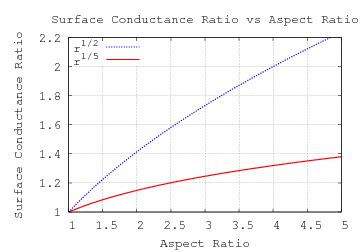A rectangle (their roof wasn't) with that area and perimeter would have an aspect-ratio of 4.8. The ratio of surface conductivities for a rectangle with aspect-ratio r along its two axis is:

 k ⋅ C ⋅ (V⋅S/ν)E Pr1/3 / S k ⋅ C ⋅ (V⋅r⋅S/ν)E Pr1/3 / (r⋅S) = r 1-E

In the turbulent case, an aspect-ratio of 2 leads to a surface conductance ratio of 1.15. That probably (and larger ratios certainly) would have been detected if wind directions were stable; so the independence of wind direction is probably due to its short-term variability.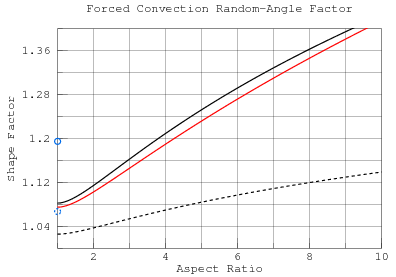The Forced Off-Axis Convection section developed formulas Ftur and Flam for turbulent and laminar rotation factors which are functions of aspect-ratio and azimuth angle in the plane of a rectangular plate using the geometric mean of the sides as characteristic length. To the right is a graph of those rotation factors (numerically) averaged over 360° of flow direction versus aspect-ratio. The laminar curve Flam is solid black; turbulent Ftur is dashed black. The red curve is an approximation to Flam as a function of area A and perimeter P of the rectangle:

 0.615 + P 8.7 A1/2 = 0.615 + A1/2 8.7 L*

Expressing the shape factor in terms of general shape metrics may make extension to other convex polygons possible. A regular polygon approaches the circle as its number of sides increases. The shape factors for a circular disk under laminar and turbulent flow are shown as solid and dashed blue circles in the figure. The turbulent disk shape factor is 4% higher than its respective square plate having the same area; the laminar disk is 10% higher.Instead of multiplying h by a shape factor, Clear et al adjust the characteristic length to account for the effect of averaging over wind direction, calling it the effective length. The chart to the right shows factors which, when multiplied by the geometric mean of the sides (A1/2) produces the effective-length Leff. The effective-length shape factors are simply Ftur−5 and Flam−2

The spread between the shape factors grows from 3% at an aspect-ratio of 1:1 to 6% at an aspect-ratio of 10:1. Formulas with Re exponents between 1/2 and 4/5 will be contained in this band. Thus a single Leff (shown in red), which stays within those bounds will approximate Re exponents between 1/2 and 4/5 well.

 Leff = A1/2 / ( 0.615 + P 8.7 A1/2 )1/2 LEAAEffective-length greatly simplifies treatment of uniformly distributed flow direction. Can it do the same for Forced Off-Axis Convection? To the right are plots of laminar and turbulent off-axis rotation factors.Laminar and turbulent effective-lengths are very close, enabling a single formula for laminar and turbulent flows and use with the scaled-Colburn-analogy asymptote.

 Leff = A1/2 |cos φ|−1 r−1/2 ( 1 + |tan φ|3 r )−2 r>|tan φ| LERR A1/2 |sin φ|−1 r+1/2 ( 1 + r3 |tan φ| )−2 0The solid lines in the graph to the right show the rotation-factors for 1 m2 rectangular plates with ε=10−4 m and aspect-ratios of 1:1 (black), 2:1 (blue), and 4:1 (red) computed by integration. The dashed lines are the rotation factors computed using Leff.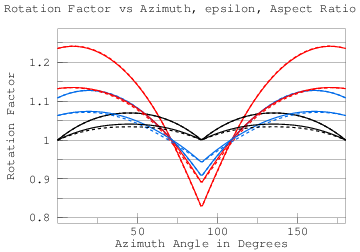The graph to the right shows the rotation-factors by integration (solid) and using effective-length (dashed) for ε=10−3 m (higher) and ε=10−5 m (lower).

So the complexities of integrating RS turn out not to matter because the convective surface conductance of the rotated plate can easily be computed using effective-length LERR.

### Natural Off-Axis Convection

So far, the natural convective analysis has assumed a rectangular plate with at least one pair of horizontal edges. This section extends the analysis to horizontal and vertical rotated plates. Rotation of the plate out of the horizontal or vertical planes has already been covered.

The three modes of (convex flat plate) natural convection use different characteristic lengths, so they must be individually treated. The upward facing mode depends on L* (area/perimeter), which is already independent of rotation as evidenced by its working for a variety of convex shapes (Lloyd and Moran).

The downward convection mode is only active when the plate is horizontal. Its characteristic-length is half of the shortest side, which is also independent of orientation.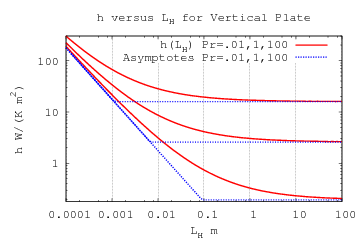The vertical plate convection mode corresponds to formula Nu':

 Nu'(Ra) = ( 0.825 + 0.387 [Ra' / Ξ(Pr)]1/6 )2

Ra' is proportional to L3. As a function of L, h' is an L1/2-norm of 0.825 k/L and a constant term. The constant term is independent of L.A rotation factor for the 0.825 k/L term can derived analogously to FTRR and FLRR, but which reduces to a simpler form:

 F'(r, φ) = |cos φ| r1/2 + |sin φ| r−1/2 FVRR

The rotation factor for the constant term is 1.

The effective length Leff is simply A1/2/F'(rφ). It can be further reduced:

 Leff = LH LW |cos φ| LW + |sin φ| LH LVRR

The graph on the left compares h'(Leff) with h'(L)⋅F', both with mean side lengths of 10−4 m. For side lengths greater than 1 m, h' is much less sensitive to Leff and rotation. The graph on the right compares h'(Leff) with h'(L), both with mean side lengths of 1 m.

 A1/2=10−4 m A1/2=1 m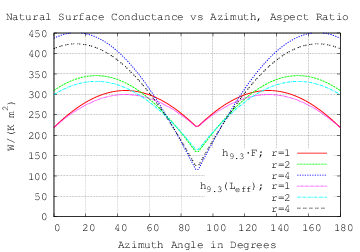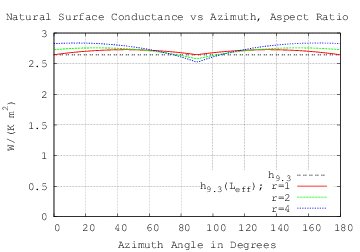## Mixed Convection

### Local Mixed Convection

In An Experimental Study of Mixed, Forced, and Free Convection Heat Transfer From a Horizontal Flat Plate to Air  X. A. Wang proposes local correlations for upward natural, mixed, and forced convection from a horizontal plate:

 Nuxf = 3+0.0253 Re0.8 for 0.068 Re2.2 > Gr (11) Nux = 2.7 ( Gr / Re2.2 )1/3 ( 3+0.0253 Re0.8 ) for 0.068 Re2.2 ≤ Gr ≤ 55.3 Re5/3 (12) Nuxe = 0.14 Gr1/3 for Gr > 55.3 Re5/3 (13)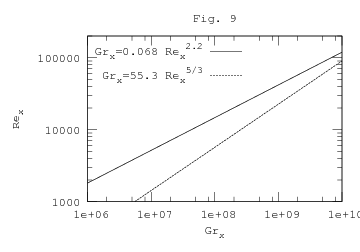Fig.9 from the paper (recreated to the right) shows that the boundaries of the mixture zone (correlation 12) will cross. Equating the constraints finds that the intersection is at Re=2.86×105 and Gr=6.87×1010. The paper gives no guidance about how to treat Re and Gr values greater than this (which are in the roof range-of-interest).

The technique for finding average h in Laminar-Turbulent Progression only works when the correlation expression tends to zero as Re(x) goes to zero. The 3 added to 0.0253 Rex4/5 prevents the integral from converging. At high Re values, formulas 11 and 12 can be approximated:

 Nuxf ≈ 0.0253 Rex4/5 Nux ≈ 0.0683 Gr1/3 Rex1/15

The Wang formulas don't express a dependence on Pr. The working fluid is air with the plate temperature ranging from 19 K to 106 K hotter than ambient. The paper doesn't reveal the ambient temperature, and describes heaters but not coolers. Taking as ambient the mean temperature of Shanghai, 16.1°C, the Pr range for Wang's experiments is 0.715. to 0.723. Incorporating Pr back into formulas 11 and 13 yields formulas within 5% of T8.10 and T9.8:

 Nuxf ≈ 0.0282 Rex4/5 Pr1/3 Nuxe ≈ 0.156 Gr1/3 Pr1/3

Why can the local Nuxe be compared with the average Nu of T9.8? The dependence of Nuxe on x is linear; so its average h is the same as its (constant) local h.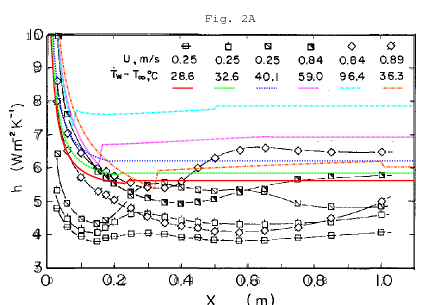The measured data is shown only in Fig. 2, plotting local h versus distance from the leading edge. To the right, the values predicted by formulas 11, 12, and 13 are plotted in colors over Wang's graph. There are discontinuities at both transitions and, for x≥0.2 m, predicted h is significantly higher than measured.

Because Wang's (local) model doesn't work, trying to derive average h formulas from its divergent integrals would be pointless. Can a de novo analysis fit Wang's experiments?The curves predicted by (forced laminar) T8.8, (forced turbulent) T8.10, and (natural turbulent) T9.8 for two cases are plotted on Fig. 2 to the right. For V=0.84, the transition from laminar to turbulent happens around x=0.075 m, at which Re=3548. This is much less than the Re=5×105 threshold given by Table 8. The low Re threshold could be due to a rough leading edge or turbulence in the impinging flow.

The two horizontal T9.8 lines are close in height to the right side of Wang's corresponding curves. But there were several problems: T9.8 should use L*=area/perimeter=0.05⋅L/(0.1+L), not the linear L that T8.8 and T8.10 use. In Wang's 0.1×1 m apparatus, L* ranges between 0 and 0.045 m. At the ΔT values from Fig.2A, Ra is bounded by 4.8×105, an order of magnitude below the range of applicability of T9.8.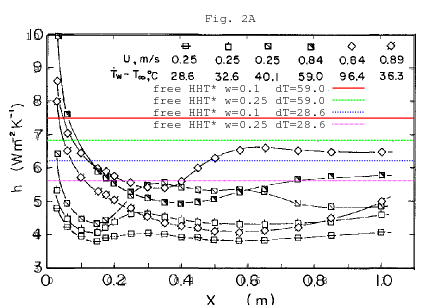Forced convection is parametrized by distance from the leading edge because the flow controls the boundary layer near the edge. Natural upward convection from a horizontal plate has flow from all edges; that is why it uses L*. The heated strip in Wang's experiment is 10 times longer than it is wide. The forced flow at the leading edge will not affect most of that length, instead behaving as an infinite strip, L*=w/2. Unfortunately, the heated strip is flanked by insulated strips 0.075 m wide on each side. These reduce the natural convective flow across the edge of the heated strip, perhaps explaining why the measured h is lower than Nu* predicts, both for 0.1 m and 0.25 m widths. The graph to the right shows Nu* at two temperatures for (fully heated) widths of 0.1 m and 0.25 m.

### Correlations From Field Measurements

An Empirical Correlation for the Outside Convective Air Film Coefficient for Horizontal Roofs by R.D. Clear, L. Gartland and F.C. Winkelmann takes a quite different approach. The roofs of two commercial buildings were instrumented with thermal and meteorological sensors (at their centers) and sampled at 15 minute intervals for over a year. Because of the poor predictive power of the sky emissivity models, they exclued cloudy days. Along with exclusions for condensation and other reasons, they used less than 1/6 of the data collected.

Because of the size of the roofs, Ra at the measurement point always exceeded the range for laminar natural convection for ΔT > 0 and, in fact, often exceeded by a factor of 100 or more the recommended range of the equation for turbulent natural convection. Re is proportional to wind speed, and there were a substantial number of low wind speed points (< 0.1 m/s) that gave Re values that were nominally in the laminar flow region. However, the fits to the data were almost always better if the flow was assumed to be turbulent. In retrospect, it seems likely that any time natural convection is turbulent, then the mixed natural/forced convection should be turbulent also. All of our fits are based on turbulent flow at the measurement point for both the natural and forced convection conditions.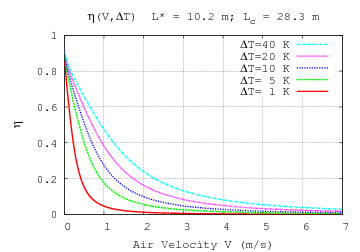C and D are the fitted constants in:

 f2(hn,hf ) ΔT = [ η C hn + D hf ] ΔT (4b)

where η is 1 for still air and tends to 0 as wind-speed increases:

 η = ln(1 + Grx / Rex2) 1 + ln(1 + Grx / Rex2)

Rex is the local Reynolds number because their wind-speed was measured at one point on the roof. The subscript of Grx implies that the Grashof Number is also local, but the formulas they used (T9.7 and T9.8) are for average surface conductance. It is possible that η requires adjustment for use with average Reynolds numbers.

Their fitted values are C=1.03 and D=1.66 with standard-error of 0.03 and 0.02, respectively.

With L*=10.2 m and ΔT=25 K, Ra is larger than 2×1012, exercising a scale of operation which is difficult to test in the laboratory. The two graphs below are of:

... a representative sample of data (July 1996, Davis) with cloudy days and condensation conditions removed, and corrected for conduction time lag between sensor location and roof surface.
We removed all data with ΔT < 0, i.e., roof temperature less than outside air temperature. This exclusion was, like the non-clear sky exclusion, due to our reliance on a Qsky estimation.
(In Figs. 8, 9 and 10, a small number of points with h < -20 or > 40 were not plotted to avoid losing detail in the remaining data.) During the day h generally increases during the morning to a mid-afternoon peak, then declines. This pattern reflects the ΔT and wind speed patterns at the site.
Figure 9 shows h vs. ΔT. The dependence on ΔT is fairly weak and we see the loss in precision as ΔT approaches zero.
Figure 10 shows h vs. wind speed. The dependence on wind speed is slightly sub-linear.Over Fig. 9 I have plotted the convective surface conductances predicted by formulas T9.8 and Nu* (natural convection above a hot horizontal plate). Above surface-to-air temperature differences of 20 K, the measured values are greater than or equal to those predicted by Nu*. This is consistent with the natural convection being aided by forced convection at low wind speeds. The forced convection contribution appears to diminish for larger temperature differences; this could be explained by the larger temperature differences being acheived only at low wind speeds (higher wind speeds cool the roof too much).

When the temperature difference is small:

... the long-wave radiative loss from the roof dominates: it is typically 10 times or more higher than the convective and conductive heat transfer. Under these conditions a small percentage error in Qsky can lead to large and systematic errors in hΔT ...

This could explain the flaring of h values below temperature differences of 10°C.

Because of the large Rayleigh numbers, Nu* is very close to T9.8. (Nu in) T9.8 is a linear function of L*; so h has no dependence on L*. Thus h at the center of the roof (where they measured it) should be the same as the average for the whole roof. The situation for forced convection is more complicated. The forced convection component at the center of the roof is local convection with characteristic length from the edge to center, rather than across the whole roof.

The characteristic length for forced convection is defined as the average distance from the roof perimeter to the heat transfer measurement point.

Their characteristic length for forced convection at the Davis site is 28.3 m. While the curve drawn on Fig. 9 is the curve for the average over the roof, the curves drawn on Fig. 10 are intended to reproduce the measurements taken at the center of the roof. The roof average will use a different L and involve T8.11 instead of T8.10 (whose ratio is 5:4).Over Fig. 10 I have plotted f2(hn,hf) from equation (4b) for D values of 1.66 and 1.33 with ΔT values of 22 K and 4.4 K, respectively.

There are only 20 points at wind-speeds greater than 4 m/s. Considered in isolation they are a strong signal for a D value of 1.33. Because there are so few points at higher wind-speeds, one might conclude that h values at wind-speeds above 4 m/s are unimportant. But looking at the histogram of wind speeds for Honolulu shows that the yearly average wind speed is greater than 4 m/s at that locale.

In discarding 86% of the points, the authors raised the average surface-to-air-temperature-difference from 4.4 K to 22 K, a fivefold increase! The removal of low ΔT points introduced a large bias into the D value that the authors fit (1.66).

Can anything be salvaged from Fig. 10? While forced convection at low wind-speed aids natural convection, natural convection does not aid forced convection at higher wind-speeds. Thus the h values for wind-speeds greater than 4 m/s should be much less affected by the culling of low ΔT points than those at lower wind-speeds.

Clear et al model the effect of surface roughness as a scale factor of Nu (and h). The h versus V curves from Surface Roughness are similar enough to support fitting a scale factor. But the authors didn't measure the roughness of the roof; someone trying to apply their results has insufficient information for predicting h without reproducing their experiment to fit the hT8.10 coefficient D.

What about mixed convection from plates which are not level upward facing? Under the heading Limitations and Applicability, Clear et al write:

Our expressions for h are applicable in the following situations:
1. The roof is horizontal. However, it is probably safe to use the formula for roof tilts up to about 20°. In no case should it be applied to vertical walls.
2. The roof is dry. The formulas should not be used when it is raining or when condensation is likely (surface temperature below dewpoint temperature).
3. The roof surface is flat and relatively unobstructed, i.e., at most a few percent of the roof area has protrusions like vents, roof-top equipment, etc.; the height of the roof parapet, if present, is only a few percent of the roof dimensions; and the roof surface is not in the wind shadow of another part of the building.

Caveat 1 rules out application of their work to vertical plates; caveat 2 rules out most downward convection.

The function η compares Gr=Ra/Pr and Re2. But in Natural Convection Summary, Nu', Nu*, and NuR all use different characteristic-lengths, and Ra being proportional to L3 magnifies these differences. Adding to the difficulty of finding an effective Gr is that for downward convection from an inclined plate one of three characteristic-lengths applies depending on the h values computed with them. Furthermore, the Ra arguments to formulas Nu' and Nu* are multiplied by cos θ and |sin θ|, respectively. Should η include these factors with Gr? The published convection literature provides no experimental basis for resolving any of these issues.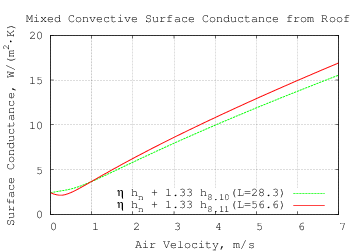Returning to horizontal plates, the graph to the right gives an expanded view of the green trace from Figure 10 above (local mixed convection). The red trace has double the forced characteric length L and uses T8.11 instead of T8.10 to model the average convective surface conductance from that roof. The dip at V=.25 m/s is due to the doubling of L (28.3 m to 56.6 m), not the switch from T8.10 to T8.11.This plot compares the red trace from the previous graph with scaled-Colburn-analogy curves for various surface roughness values (ε). Because of surface roughness, all but the lowest scaled-Colburn-analogy trace are nearly straight above 1 m/s; the lowest trace and the scaled T8.11 trace in red are proportional to Re4/5 above 1 m/s.

Consider the natural upward convection from a heated horizontal plate with a forced laminar flow parallel to the plate. Each (laminar) layer is shifted in the direction of the flow, the shift increasing with the layer's height above the plate. The relative shifting of the layers slightly increases the mixing of hotter fluid with cool fluid, increasing the heat transferred from plate to fluid. Therefore the surface conductance should be monotonically increasing with air velocity; so the η function, which produces a local minimum in the graphs above, doesn't appear to correctly model average surface conductance in laminar flows.

Forced turbulent flow erodes the laminar boundar layer, which also increases the surface conductance. Thus mixed convective surface conductance should increase monotonically with fluid speed.

### Mixed Convection from a Rough Plate

My article Mixed Convection from a Rough Plate develops a theory of convection for rough plates at any inclination and tests it against measurements from the Convection Machine. The Convection Machine plate with 3 mm of roughness is so rough that it doesn't explore the transition between turbulent flow from a smooth plate (Re4/5) and turbulent flow from the rough plate (linear Re). It will be modified to do so. In the meantime, I will assume that the two modes are in simple competition:

 Nu8.11= 0.037 Re4/5 Pr1/3 (T8.11) NuRT = Re Pr1/3 32 log10( ε / L )2 εLF < 0.085 Re1/5 RT
 hF = k⋅max(NuRT(Re), Nu8.11(Re))/LF

Allowing for the plate to be rotated φ around its center adds more complication, which is detailed below. Reff is the effective-length for natural-convection from the plate.

### Mixed Convection Summary

Proposed is the complete convective surface conductance for one side of a flat LH by LW rectangular isothermal plate rotated φ around the center of the plate (in its plane) and inclined θ from vertical (θ<0 is facing upward) and with (forced) bulk fluid velocity is Vf at angle ψ from the normal projection of vertical in the plane of the plate.

 Pr = cp⋅μ/k

Shape Parameters

 A = LW LH r = LW / LH L* = A 2 (LW+LH) R = min(LH, LW)/2 Reff = LH LW |cos φ| LW + |sin φ| LH ‖x,y‖p = (xp+yp)1/p x ≥ 0; y ≥ 0; p > 0 θe = θ TS≥TF −θ TS

Natural Convection

 Ra* = β⋅|ΔT|⋅g⋅L*3 ν2 Pr |sin θe| RaV = β⋅|ΔT|⋅g⋅Reff3 ν2 Pr |cos θe| RaR = β⋅|ΔT|⋅g⋅R3 ν2 Pr |sin θe|
 NuNu* = {0.671 + 0.370 Ra*1/6}2 −90°≤θe≤0° 1≤Ra*≤1012 Nu' = {.825 + .387 RaV1/6 [1+(.492/Pr)9/16]8/27 }2 1≤RaV≤1012 NuR = 0.544 RaR1/5 [1+(0.785/Pr)3/5]1/3 0°≤θe≤+90°

The (forced) bulk fluid velocity is Vf at angle ψ from the normal projection of vertical in the plane of the plate.

The effective (characteristic) length for flow at angle ψ from the rectangle orientation is:

 Leff = A1/2 |cos ψ|−1 r−1/2 ( 1 + |tan ψ|3 r )−2 r>|tan ψ| LERR A1/2 |sin ψ|−1 r+1/2 ( 1 + r3 |tan ψ| )−2 0

Reynolds number for forced-convection:

 Re = V⋅Leff / ν

The forced component Nusselt number:

 Nu8.11= 0.037 Re4/5 Pr1/3 (T8.11) NuRT = Re Pr1/3 32 log10( ε / Leff )2 εLeff < 0.085 Re1/5 RT
 NuF = max(NuRT(Re), Nu8.11(Re))

The mixed convective surface conductance is:

 M(hF, hN, ψ) = max(0, cos ψ) 4hF2hN2 hF4+4hN4 −min(0, cos ψ) 16hN16 hF16+16hN16
 B(hF, hN, ψ) = {[hF2+hN2]2−2hF2hN2M(hF, hN, ψ)}1/4
 h = k⋅max( B( NuF Leff , NuR R , ψ ) , ‖ NuV LV , NuF Leff ‖2) sin θe≥0 h = k⋅max( B( NuF Leff , NuNu* L* , ψ ) , ‖ NuV LV , NuF Leff ‖2) sin θe≤0

The heat flow is h⋅A⋅ΔT.

## Application to Roofs

### Wind

A rotating cup anemometer at 10 m height is the standard wind-speed measuring instrument for the Automated Surface Observing System (ASOS); only the horizontal component of wind velocity is reported. There are formulas for estimating wind at lower heights. The important wind properties for convection are those near the roof surface. Wind speed and direction over the roof will be affected the shape and openings of the building; the most accurate modeling will require simulating airflow around the building.

For the low-pitch roofs examined here, the wind-speed, scaled for its height above terrain, should be a good estimate for the airspeed at the roof surface.

The range table for h predicts (using correlations RS and T8.11) that, for a 1 m square plate, 2 mm surface roughness can produce a forced convective surface conductance three times that of a smooth plate. In locations where ambient temperatures can exceed 45°C, a smooth roof may be preferable to a rough one in order to reduce wind-driven roof heating on very hot days.

### Flat Roofs

The simplest roofs are flat, either horizontal or slightly inclined. These are handled directly by the model. θ will be (slightly) greater than or equal to −90°.

Some horizontal flat roofs have low walls around their perimeters. This will reduce the forced convective surface conductance. As noted in the section Natural Convection from a Rough Plate, most of the inflow comes from above the plate, not along its surface. So the reduction of natural convective surface conductance may not be large.

Four Java methods in in Flat_roof.java calculate the mixed convection from a rough or smooth surfaced flat (rectangular) roof at given orientation, wind-direction and speed, temperature, air-pressure, and relative-humidity.

data-typenamereturn-unitsdescription
double`flatRoofConvect`Wheat flow
double`flatRoofHeatFlux`W/m2heat flux
double`flatRoofH`W/(K⋅m2)convective surface conductance
double`flatRoofHa`W/Kheat flow per degree of temperature difference

They all take the same arguments:

data-typeargumentunitsdescription
doubleh__mmlength
doublew__mmwidth
doubleeps__mmmean height of surface roughness
doublephi__deg°rotation around center in plane of roof
doubletheta__deg°inclination from vertical around width edge; negative is upward facing
doublepsi__deg°forced flow angle from width edge in plane of roof
doublev_f__m_per_sm/sforced bulk flow velocity
doublet_a__kKambient (bulk) air temperature
doublet_s__kKroof surface temperature
doublep__paPabulk air pressure
doublerhrelative humidity

### Peaked RoofsConsider a symmetrical peaked roof composed of two rectangular plates joind at the ridge. In the example to the right (top), each section has an 8 m span, a 2 m rise, and is 12 m long.

No rotation in the plane of the plates (which are not necessarily coplanar) is allowed, so φ=0. One of the constraints on the peaked roof model is that it should become identical to the Mixed Convection Summary model when θ=−90 (because the roof would then be flat).

−90≤θ<−60 is the inclination from vertical; the roof pitch is 90+θ (between 0° and 30°). LW is the length of the roof ridge and LH is the distance between the lower (parallel) edges of the roof.

 RH = LH |sin θ| A = LW RH r = LW / RH R = min(RH, LW)/2 Reff = RH/2 L* = A 2 (LW+RH) θe = θ TS≥TF −θ TS 0

A=LWLH/|sin θ| is the surface area of the roof and is the area by which h is multiplied.

For downward convection (roof cooler than air) Nu' flow from the ridgeline will dominate NuR flow when the roof is not flat. The effective plate dimensions for NuR will be LW by LH when θ=−90 and are uncritical otherwise. The characteristic length for Nu' flow is Reff=RH/2.

Natural Convection

 Ra* = β⋅|ΔT|⋅g⋅L*3 ν2 Pr |sin θe| RaV = β⋅|ΔT|⋅g⋅Reff3 ν2 Pr |cos θe| RaR = β⋅|ΔT|⋅g⋅R3 ν2 Pr
 NuNu* = {0.671 + 0.370 Ra*1/6}2 −90°≤θe≤0° 1≤Ra*≤1012 Nu' = {.825 + .387 RaV1/6 [1+(.492/Pr)9/16]8/27 }2 1≤RaV≤1012 NuR = 0.544 RaR1/5 [1+(0.785/Pr)3/5]1/3 0°≤θe≤+90°

The effective (characteristic) length for the mixed flow is independent of the sign of ψ. So in both cases it is:

 Leff = A1/2 |cos ψ|−1 r−1/2 ( 1 + |tan ψ|3 r )−2 r>|tan ψ| LERR A1/2 |sin ψ|−1 r+1/2 ( 1 + r3 |tan ψ| )−2 0

Reynolds number for forced-convection:

 Re = V⋅Leff / ν

The forced component Nusselt number:

 Nu8.11= 0.037 Re4/5 Pr1/3 (T8.11) NuRT = Re Pr1/3 32 log10( ε / Leff )2 εLeff < 0.085 Re1/5 RT
 NuF = max(NuRT(Re), Nu8.11(Re))

The mixed convective surface conductance is:

 h = k⋅max(‖ NuR R , NuF Leff ‖4 , ‖ NuV LV , NuF Leff ‖2) sin θe≥0 h = k⋅max(‖ NuNu* L* , NuF Leff ‖2 , ‖ NuV LV , NuF Leff ‖2) sin θe≤0

The heat flow is h⋅A⋅ΔT =h⋅ΔTLWLH/|sin θ|.

Four Java methods in in Peaked_roof.java calculate the mixed convection from a rough or smooth surfaced symmetrical peaked roof at given wind-direction and velocity, temperature, air-pressure, and relative-humidity.

data-typenamereturn-unitsdescription
double`peakedRoofConvect`Wheat flow
double`peakedRoofHeatFlux`W/m2heat flux
double`peakedRoofH`W/(K⋅m2)convective surface conductance
double`peakedRoofHa`W/Kheat flow per degree of temperature difference

They all take the same arguments:

data-typeargumentunitsdescription
doubleh__mmlength
doublew__mmwidth
doubleeps__mmmean height of surface roughness
doubletheta__deg°inclination from vertical; −90≤θ<−60
doublepsi__deg°forced flow angle from ridge in horizontal plane
doublev_f__m_per_sm/sforced bulk flow velocity
doublet_a__kKambient (bulk) air temperature
doublet_s__kKroof surface temperature
doublep__paPabulk air pressure
doublerhrelative humidity

The convecting surfaces of the peaked roof are convex when considered in combination. Two rectangular plates meeting at a lower height than their centers is not convex and their analysis is not addressed by current theory. For a valley roof, unless the joined edge is short compared with the other dimension, the Nu' mode will be significantly reduced because of the extra distance air must travel to enter or exit near the joined edge.

### Roof Performance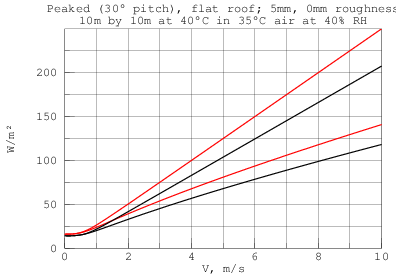The graph to the right compares heat flux from a flat level roof (black) with that from a symmetrical peaked roof with 30° pitch (red). The upper two traces are with 5 mm mean height of roughness; the lower two traces for a smooth surface. All are for roofs covering a 10 m by 10 m building with the roof 5°C hotter than ambient.

The wind direction is diagonal across the roof. At wind-speeds greater than 4 m/s the surface roughness has greater effect on heat-flow than the roof configuration.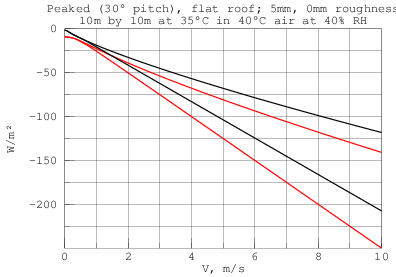The graph to the right shows the heat flux from the same roofs, but with the roofs 5°C cooler than ambient. The peaked roof's NuR mode is overwhelmed by Nu' in still air, so its downward natural convection does not get as close to 0 as the flat plate does.

## Conclusion

A complete mixed single-phase convection model has been presented for flat rectangular plates. Its novel features are:

• natural convection from a rectangular plate in any orientation,

• a formula for forced convection over a rough flat surface,

• correspondence between forced convection and the vertical-plate mode of natural convection,

• vector sum of forced-equivalent velocity and forced velocity vectors,

• competition between convective modes, and

• L4-norm combination of natural convective modes.

## Future Work

### Flat Convex Plates

The model could be extended to arbitrary convex shapes by extending each of the convective component modes to arbitrary shapes. The shape-factor for both the forced and vertical-plate modes can be computed by integration as was done in Forced Off-Axis Convection and Natural Off-Axis Convection.

The upward convective mode (Nu*) is a function of L*, which is defined for all flat convex shapes. Lloyd and Moran find the formula accurate for a variety of convex shapes.

The challenge is finding the characteristic-length for downward convection from a horizontal plate. Downward convection from a rectangular plate self-organizes into two rollers parallel to a longest edge and has characteristic-length, R, which is half of the shorter edge. Schulenberg gives a separate correlation (47) for downward convection from a disk which has the same exponents as correlation (45) for an infinite strip. Through appropriate choice of characteristic-length R, can these two correlations be unified?

### Convection with Phase-Change

Convection in fluids which can undergo phase changes is of great practical interest in the case of water-vapor condensation on roofs. While there is a body of theory and experiment dealing with condensation from a pure phase-change fluid (eg. steam), there is less available dealing with condensation from a mixture of condensing and non-condensing gases (eg. air).

### Experiments

#### Measure the dependence of Nu/Pr1/3 on Re and relative roughness 0<ε≪L.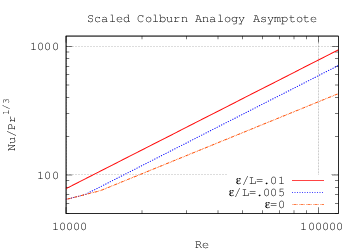We know that for small Reynolds number flow over low surface roughness, the convective surface conductance is close to that of a smooth plate. So only strong turbulent flow over a rough surface needs to be measured. Not needing laminar flow simplifies the wind tunnel requirements considerably.

The table below shows the dimensionless quantities that the scaled-Colburn-analogy-asymptote model predicts for plates with relative roughnesses of 0.01, 0.005, and 0 (smooth).

ε/L Re Nu/Pr1/3 Re Nu/Pr1/3 Re Nu/Pr1/3 0.0100 2.00×104 1.56×102 4.00×104 3.12×102 8.00×104 6.25×102 0.0050 2.00×104 1.18×102 4.00×104 2.36×102 8.00×104 4.72×102 0.0000 2.00×104 1.02×102 4.00×104 1.78×102 8.00×104 3.09×102

This measurement has been performed by the Convection Machine for ε/L=0.01 between Re=5000 and Re=100000; the results appear to confirm R8.11.

#### Determine if surface roughness affects turbulent natural convection of a vertical rectangular plate.

The reasoning that natural vertical convection is insensitive to surface roughness might not hold at high Rayleigh numbers.

The plate described in Convection Measurements is not large enough to reach turbulent natural convection in air with a practical temperature difference (<50K).

#### Measure the dependence of natural surface conductance h on orientation of a rectangular plate.

Because the natural convection arising from the the plate described in Convection Measurements is expected to be laminar, it can be used to do this measurement. However, the apparatus designed for testing the higher heat transfer rates of forced convection will suffer from long settling times measuring natural convection.

#### Measure the surface conductance h of a plate (in various orientations) at natural-to-forced transition flows.

This would require stable laminar low-speed flow in the wind tunnel, which can be difficult to achieve and measure accurately.

## Bibliography

1. 2009 ASHRAE Fundamentals Handbook (SI)
American Society of Heating Refrigerating and Air-conditioning Engineers Inc.,
ISBN: 978-1-931862-70-7 (I-P); 978-1-931862-71-4 (SI)
ISSN: 1523-7222 (I-P); 1523-7230 (SI)

2. The Electronics Handbook, Second Edition,
Editor: Jerry C. Whitaker,
Technical Press, Morgan Hill, California, USA, 2005
ISBN: 9780849318894

3. Kyle A. Brucker, Joseph Majdalani,
Effective thermal conductivity of common geometric shapes,
International Journal of Heat nd Mass Transfer,
Volume 48, Issues 23-24, November 2005, Pages 4779-4796,
ISSN 0017-9310, DOI: 10.1016/j.ijheatmasstransfer.2005.05.007.

4. R. J. Goldstein, E. M. Sparrow, D. C. Jones,
Natural convection mass transfer adjacent to horizontal plates,
International Journal of Heat and Mass Transfer,
Volume 16, Issue 5, May 1973, Pages 1025-1035,
ISSN 0017-9310, DOI: 10.1016/0017-9310(73)90041-0.

5. J. R. Lloyd and W. R. Moran,
Natural Convection Adjacent to Horizontal Surface of Various Planforms,
J. Heat Transfer 96, 443-447 (1974),
DOI:10.1115/1.3450224

6. Stuart W. Churchill, Humbert H. S. Chu,
Correlating equations for laminar and turbulent free convection from a vertical plate,
International Journal of Heat and Mass Transfer,
Volume 18, Issue 11, November 1975, Pages 1323-1329,
ISSN 0017-9310,
DOI: 10.1016/0017-9310(75)90243-4

7. Tetsu Fujii, Hideaki Imura,
Natural-convection heat transfer from a plate with arbitrary inclination,
International Journal of Heat and Mass Transfer,
Volume 15, Issue 4, April 1972, Pages 755-764,
IN5-IN6, 765-767, ISSN 0017-9310, DOI: 10.1016/0017-9310(72)90118-4.

8. T. Schulenberg,
Natural convection heat transfer below downward facing horizontal surfaces,
International Journal of Heat and Mass Transfer,
Volume 28, Issue 2, February 1985, Pages 467-477,
ISSN 0017-9310, DOI: 10.1016/0017-9310(85)90080-8.

9. X. A. Wang,
An Experimental Study of Mixed, Forced, and Free Convection Heat Transfer From a Horizontal Flat Plate to Air,
J. Heat Transfer,
Volume 104, Issue 1, February 1982, Pages 139–144,
DOI: 10.1115/1.3245040.

10. Automated Surface Observing System (ASOS) User's Guide,
Department of Defense
United States Navy
March 1998
http://www.nws.noaa.gov/asos/

11. E. M. Sparrow and K. K. Tien,
Forced Convection Heat Transfer at an Inclined and Yawed Square Plate—Application to Solar Collectors
J. Heat Transfer,
Volume 99, November 1977, Issue 4, 507 (6 pages)
DOI: 10.1115/1.3450734.

12. C. P. Kothandaraman,
Fundamentals of Heat and Mass Transfer,
Third Edition, 2006,
New Age International (P) Limited, Publishers, New Delhi

13. R.D. Clear, L. Gartland and F.C. Winkelmann,
An Empirical Correlation for the Outside Convective Air Film Coefficient for Horizontal Roofs,
Environmental Energy Technologies Division,
Lawrence Berkeley National Laboratory,
LBNL-47275 January 2001

14. S Beck and R Collins, University of Sheffield,
File:Moody diagram.jpg,
Wikipedia, the free encyclopedia, 2008,

15. Rowley, F.B., Algren, A.B., and Blackshaw, J.L.,
Surface Conductances as Affected by Air Velocity, Temperature and Character of Surface,
ASHVE Trans. 36, p 429 (1930).

16. John H. Lienhard IV and John H. Lienhard V,
A heat transfer textbook, 4th edition, 2011,
Phlogiston Press, Cambridge, Massachusetts, US
TJ260.L445

17. Churchill, Stuart W.
A comprehensive correlating equation for forced convection from flat plates,
AIChE Journal, Volume 22, Issue 2, 1975,
DOI: 10.1002/aic.690220207

18. C. F. Colebrook and C. M. White
Experiments with Fluid Friction in Roughened Pipes,
Proc. R. Soc. Lond. A 1937 161, 367-381,
DOI: 10.1098/rspa.1937.0150

19. Moody, L. F.,
Friction factors for pipe flow,
Transactions of the ASME 66 (8): 671–684 (1944)

20. Latif M. Jiji,
Heat Convection,
Springer-Verlag Berlin Heidelberg,
ISBN 978-3-642-02970-7

21. H. T. Lin, W. S. Yu, C. C. Chen,
Comprehensive correlations for laminar mixed convection on vertical and horizontal flat plates,
Heat and Mass Transfer, Vol. 25, No. 6. (1 November 1990), pp. 353-359.,
doi:10.1007/BF01811559 Key: citeulike:8824378

22. P. T. Tsilingiris,
Thermophysical and transport properties of humid air at temperature range between 0 and 100°C,
Energy Conversion and Management,
Volume 49, Issue 5, May 2008, Pages 1098-1110,
ISSN 0196-8904, http://dx.doi.org/10.1016/j.enconman.2007.09.015.

23. Z. K. Morvay, D. D. Gvozdenac,
Applied Industrial Energy and Environmental Management,
Toolbox 6: Thermodynamic and Transport Properties of Moist Air,
John Wiley & Sons, Ltd

24. K. Kadoya, N. Matsunaga, and A. Nagashima,
Viscosity and Thermal Conductivity of Dry Air in the Gaseous Phase,
J. Phys. Chem. Ref. Data 14, 947 (1985),
http://dx.doi.org/10.1063/1.555744 (24 pages)

25. A. Wexler,
Vapor pressure formulation for water in range 0 to 100°C. A revision (1976),
J. Res. Nat. Bur. Stand. Sec. A: Phys. Ch., Vol. 80A, Nos. 5&6, p. 775,
http://archive.org/details/jresv80An5-6p775

Copyright © 2011, 2012, 2013, 2014, 2015, 2016, 2019, 2020 Aubrey Jaffer

 I am a guest and not a member of the MIT Computer Science and Artificial Intelligence Laboratory.  My actions and comments do not reflect in any way on MIT. SimRoof agj @ alum.mit.edu Go Figure!